Equilibrium Constant for Heterogeneous Equilibria - Chemical Equilibrium

# Equilibrium Constant for Heterogeneous Equilibria - Chemical Equilibrium Notes | Study Physical Chemistry - Chemistry

## Document Description: Equilibrium Constant for Heterogeneous Equilibria - Chemical Equilibrium for Chemistry 2022 is part of Chemical Equilibrium for Physical Chemistry preparation. The notes and questions for Equilibrium Constant for Heterogeneous Equilibria - Chemical Equilibrium have been prepared according to the Chemistry exam syllabus. Information about Equilibrium Constant for Heterogeneous Equilibria - Chemical Equilibrium covers topics like and Equilibrium Constant for Heterogeneous Equilibria - Chemical Equilibrium Example, for Chemistry 2022 Exam. Find important definitions, questions, notes, meanings, examples, exercises and tests below for Equilibrium Constant for Heterogeneous Equilibria - Chemical Equilibrium.

Introduction of Equilibrium Constant for Heterogeneous Equilibria - Chemical Equilibrium in English is available as part of our Physical Chemistry for Chemistry & Equilibrium Constant for Heterogeneous Equilibria - Chemical Equilibrium in Hindi for Physical Chemistry course. Download more important topics related with Chemical Equilibrium, notes, lectures and mock test series for Chemistry Exam by signing up for free. Chemistry: Equilibrium Constant for Heterogeneous Equilibria - Chemical Equilibrium Notes | Study Physical Chemistry - Chemistry
 1 Crore+ students have signed up on EduRev. Have you?

Equilibrium Constant for Heterogeneous Equilibria
The equilibrium which involves reactants and products in different physical states. The law of mass action can also be applied on heterogenous equilibria as it was applied for homogenous equilibria (involving reactants and products in same physical states).
(i) Thermal Dissociation of Solid Ammonium Chloride:
The thermal dissociation of NH4Cl(s) takes place in a closed container according to the equation

NH4Cl(s) ⇌ NH3(g) + HCl(g)

Let us consider 1 mole of NH4Cl(s) is kept in a closed container of vo lume ‘V’ litre at temperature TK and if x mole of NH4Cl dissociates at equilibrium then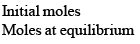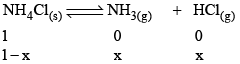Applying law of mass action,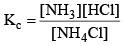As NH4Cl is a pure solid, so there is no appreciable change in its concentration. Thus,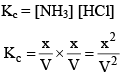And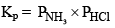(ii) Thermal Dissociation of Ag2CO3:

Ag2CO3(s) dissociates thermally according to the equation.

Ag2CO3(s) ⇌ Ag2O(s) + CO2(g)

Applying law of mass action, at constant temperature, we get,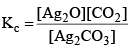Now, let us consider that 1 mole of Ag2CO3(s) is heated in a closed container of volume V and x mol of Ag2CO3(s) dissociates at equilibrium, then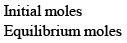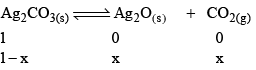Now, as Ag2CO3 and Ag2O are solids, so their concentration can be assumed to be constants
Thus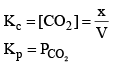7. Significance of magnitude of equilibrium constant:
· A very large value of Kc or Kp signifies that the forward reactions has gone to completion or very nearly so.
· A very small value of Kc or Kp signifies that the forward reaction has not occur to any significant extent.
· If the numerical value of Kc or Kp is neit her very large or very small, a reaction is mo st likely to reach a state of equillibrium in which both reactants and products are present.

8. Predictions of direction of the reaction: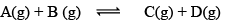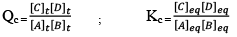QC is called React ion Quotient:
QC is the ratio of concentration of products and reactants each raised to their schoitiometric coefficient as in balanced chemical equation. Hence, three cases arises:

Q< KC  Forward direction
QC > KC  Backward direction
QC = KC  Equilibrium

Note: While dealing with KP and α, take init ial moles of reactant as 1 because ult imately they will be cancelled out.

9. Extent of Reaction:
Magnitude of equilibrium constant tells us the extent of reaction. It resembles the extent by which the reactant has been reacted or product has been formed at equilibrium.

Case I: If equilibrium constant (say KC) is very-very small.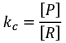(Concentration of product is very low and concentration of reactant is very high)

It clearly explains that numerator is very small and denominator is very-very high.

Hence, by this we can infer that reaction has a very low approach in forward direction and reactions has just started.

Case II: If equilibrium constant (i.e. KC) is very-very high.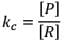(Concentration of product is very high and concentration of reactant is very low).

Hence, it explains that reaction has almost completed.

Illustration: A(g) ⇌B(g) + C(g)

Initial moles of reactant is 1 mole and volume of the container is 1L. Calculate the moles of B(g) at Equilibrium Kc = 10–50

Solution: Since the value of KC is very low.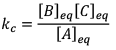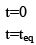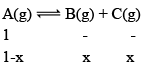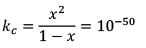Since,  x <<<<1
Hence, KC = x2 = 10–50

x = 10–25

Illustration: If initially 1 mole of all the species present one taken in 10 L closed vessel, find equilibrium concentration of each species.

A(g) ⇌ B(g) +C(g) kc = 10–2

Solution: A(g) ⇌ B(g) + C(g)

At any time concentration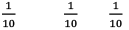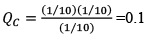Since QC > KC, so reaction will move in backward direct ion to attain equilibrium.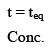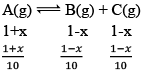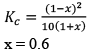Equilibrium concentration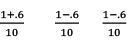Equilibrium concentration of A(g) = 0.16
Equilibrium concentration of B(g) = 0.04
Equilibrium concentration of C(g) = 0.04

Illustration: Calculate KP for the reaction

N2O4(g) ⇌ 2 NO2(g)

When NO2 is 30% dissociated and total pressure at equilibrium is 2 bar.

Solution:

Given, α = 0.3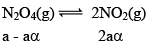We are dealing with KP and α. Hence init ial mole of N2O4 = 1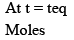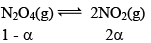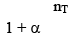Partial Pressure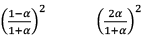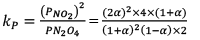Put α = 0.3
KP = 0.79

10. Dealing with degree of dissociation (α)

In terms of moles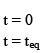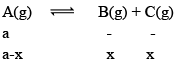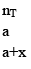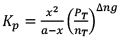In terms of α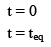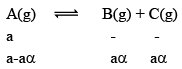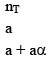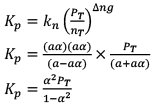The document Equilibrium Constant for Heterogeneous Equilibria - Chemical Equilibrium Notes | Study Physical Chemistry - Chemistry is a part of the Chemistry Course Physical Chemistry.
All you need of Chemistry at this link: Chemistry

## Physical Chemistry

83 videos|106 docs|31 tests
 Use Code STAYHOME200 and get INR 200 additional OFF

## Physical Chemistry

83 videos|106 docs|31 tests

Track your progress, build streaks, highlight & save important lessons and more!

,

,

,

,

,

,

,

,

,

,

,

,

,

,

,

,

,

,

,

,

,

;